Get instant live expert help with Excel or Google Sheets“My Excelchat expert helped me in less than 20 minutes, saving me what would have been 5 hours of work!”

#### Post your problem and you’ll get Expert help in seconds.

Your message must be at least 40 characters
Our professional Expert are available now. Your privacy is guaranteed.

# How to Build a Dynamic Workbook Reference in Excel

We can build a dynamic workbook reference by using the INDIRECT FUNCTION. This will enable us to easily work on different workbooks, allowing us to switch between them frequently to check and retrieve values from several worksheets.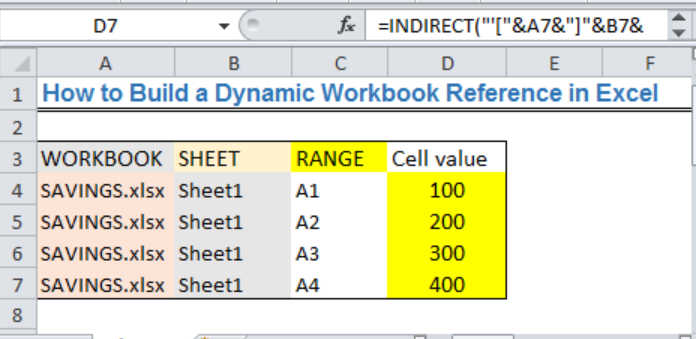Figure 1: Created Dynamic Workbook Reference

## General Formula

`=INDIRECT ("'["&workbook name&"]"&sheet name&"'!"&reference cell)`

## Formula

`=INDIRECT("'["&A2&"]"&B2&"'!"&C2)`

## Setting up the Data

• We will have two workbooks. The first is the reference workbook where we intend to create the dynamic reference in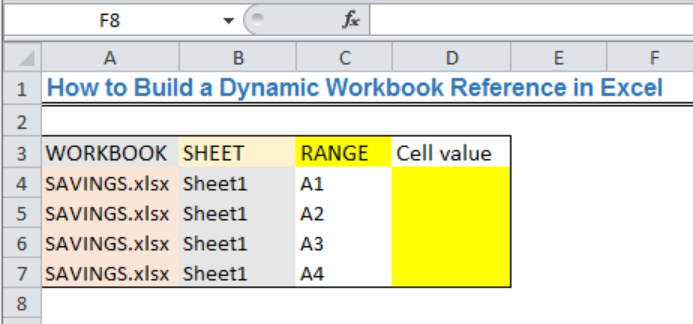Figure 2: Reference workbook

• We will name the second workbook as SAVINGS
• We will input the values into Cell A1 to Cell A4 as shown in figure 3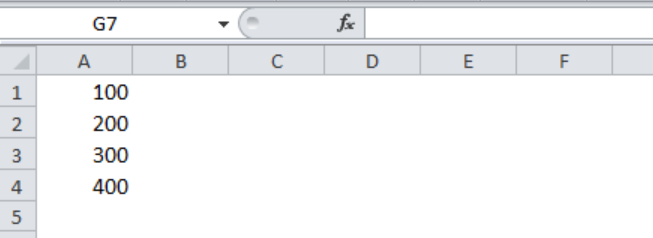Figure 3: Data contained in the second workbook titled SAVINGS

You will notice in figure 2 that the WORKBOOK has .xlsx attached to the name of the second workbook. Always add .xlsx to the name to ensure that it is a valid reference. Also, you will notice that Sheet1 in figure 3 has no space. That is why we mustn’t leave a space when inserting the data in the reference workbook in figure 2.

## Creating the Dynamic Workbook Reference

• We will click on Cell D4 of the reference workbook and insert the formula below

`=INDIRECT("'["&A4&"]"&B4&"'!"&C4)`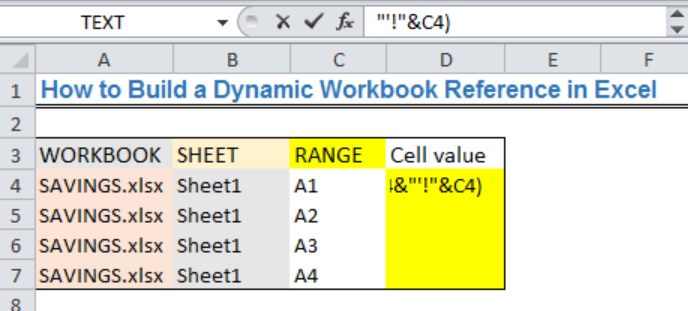Figure 4: Creating the Dynamic Workbook Reference

• We will press the enter key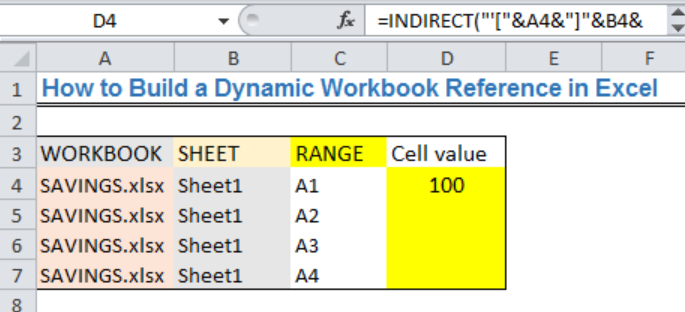Figure 5: Creating the Dynamic Workbook Reference

• We will use the drop-down feature to get the reference values for the other cells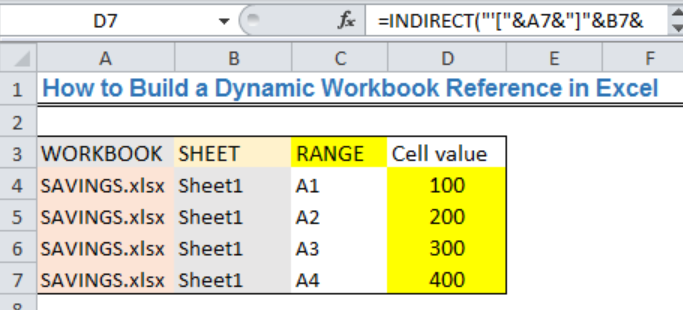Figure 6: Created Dynamic Workbook Reference

## Explanation

`=INDIRECT ("'["&workbook name&"]"&sheet name&"'!"&reference cell)`

In this formula, we want to use the INDIRECT function to create a complete reference to a specified range in the second workbook. The INDIRECT function converts text to an actual reference and returns the value in the specified cells and worksheets.

A reference to the second worksheet will look like this:

`'[SAVINGS.xlsx]Sheet1'!A4`

## Note

The workbook (SAVINGS workbook) that we intend to reference must be open, else, INDIRECT will display a #REF error.

## Instant Connection to an Expert through our Excelchat Service

Most of the time, the problem you will need to solve will be more complex than a simple application of a formula or function. If you want to save hours of research and frustration, try our live Excelchat service! Our Excel Experts are available 24/7 to answer any Excel question you may have. We guarantee a connection within 30 seconds and a customized solution within 20 minutes.

### Did this post not answer your question? Get a solution from connecting with the expert.Another blog reader asked this question today on Excelchat:
Solution examplesI have a google sheet that takes information from a google form, that creates a row every time a form is submitted. Since it is a new row that is created, rather than filling in the next row down, the formulas I have applied to some of the columns do not work. The same is true when I try and link the data to another sheet to bypass this problem. This is probably quite a general functionality issue so I won't send the actual document but let me know if you need it
Solved by F. F. in 13 minsHello, I need help regarding indirect formula of excel. 1. What is the purpose of using this formula? 2. If we use this formula with sum formula, then why we are using this with sum formula, we can also perform addition from different sheets without indirect formula ?
Solved by O. D. in 21 minsI want to dynamic access a sheet/cell from a closed file using INDEX. But when I try to "&A3&" for sheet name I forces me to select a file and then populates the sheet name instead of making it dynamically INDEX('/Users/me/Desktop/["&A2&"]"&A3&"'!A:A,1,1)
Solved by G. H. in 20 minsI need explanation for the excel formula below... =IF(ISERROR(SUMIFS(INDIRECT("'"&BE\$6&"'!"&"\$G:\$G"),INDIRECT("'"&BE\$6&"'!"&"\$R:\$R"),\$A39,INDIRECT("'"&BE\$6&"'!"&"\$F:\$F"),"<>52711",INDIRECT("'"&BE\$6&"'!"&"\$C:\$C"),10)),0,SUMIFS(INDIRECT("'"&BE\$6&"'!"&"\$G:\$G"),INDIRECT("'"&BE\$6&"'!"&"\$R:\$R"),\$A39,INDIRECT("'"&BE\$6&"'!"&"\$F:\$F"),"<>52711",INDIRECT("'"&BE\$6&"'!"&"\$C:\$C"),10))+IF(ISERROR(SUMIFS(INDIRECT("'"&BE\$4&"'!"&"\$A\$30:\$EO\$30"),INDIRECT("'"&BE\$4&"'!"&"\$A\$4:\$EO\$4"),BE\$5,INDIRECT("'"&BE\$4&"'!"&"\$A\$5:\$EO\$5"),\$A39)),0,SUMIFS(INDIRECT("'"&BE\$4&"'!"&"\$A\$30:\$EO\$30"),INDIRECT("'"&BE\$4&"'!"&"\$A\$4:\$EO\$4"),BE\$5,INDIRECT("'"&BE\$4&"'!"&"\$A\$5:\$EO\$5"),\$A39))+IF(ISERROR(SUMIF(INDIRECT("'"&BE\$6&" "&\$A\$31&"'!"&"H:H"),\$A39,(INDIRECT("'"&BE\$6&" "&\$A\$31&"'!"&"I:I")))),0,SUMIF(INDIRECT("'"&BE\$6&" "&\$A\$31&"'!"&"H:H"),\$A39,(INDIRECT("'"&BE\$6&" "&\$A\$31&"'!"&"I:I"))))
Solved by V. A. in 20 mins## Subscribe to Excelchat.coAnother blog reader asked this question today on Excelchat: